Next: Termination Test. Up: The Trust-Region subproblem Previous: How to find a   Contents

# The Rayleigh quotient trick

Ifis symmetric and the vector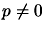, then the scalar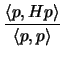is known as the Rayleigh quotient of p. The Rayleigh quotient is important because it has the following property: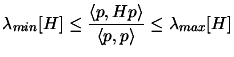(4.21)

During the Cholesky factorization of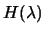, we have encountered a negative pivot at the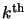stage of the decomposition for some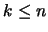. The factorization has thus failed (is indefinite). It is then possible to add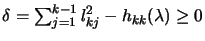to thediagonal ofso that the leading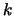bysubmatrix of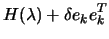is singular. It's also easy to find a vector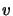for which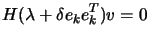(4.22)

using the Cholesky factors accumulated up to step. Setting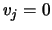for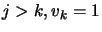, and back-solving: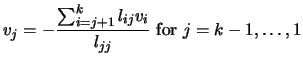gives the required vector. We then obtain a lower bound on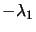by forming the inner product of 4.24 with, using the identity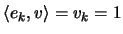and recalling that the Rayleigh quotient is greater then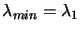, we can write: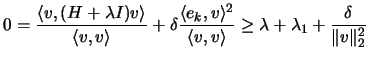This implies the bound on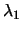: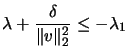In the algorithm, we set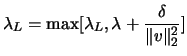Next: Termination Test. Up: The Trust-Region subproblem Previous: How to find a   Contents
Frank Vanden Berghen 2004-04-19